# Free video lessons for Foundation to Grade 8 students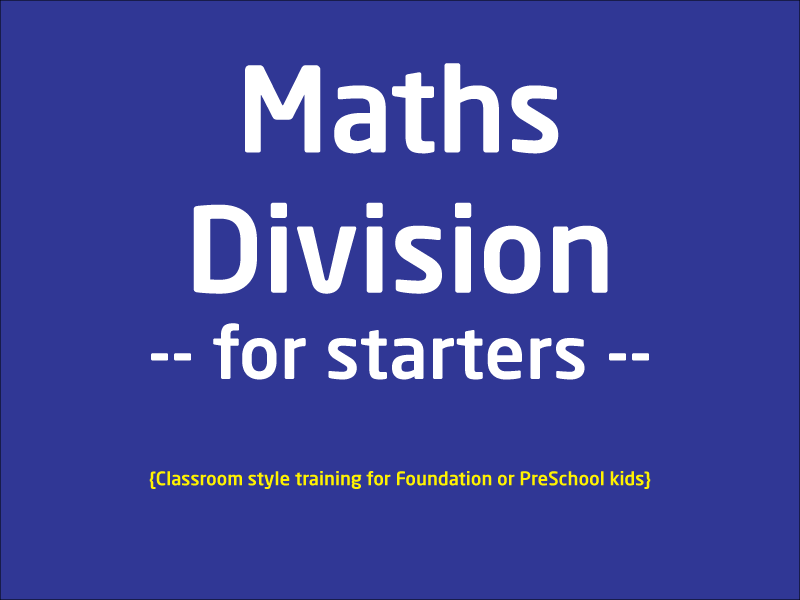### Maths division - The Basics

This lesson is a half an hour lesson explaining kids how to divide basically. This lesson does not cover remainder because that would make things too complicated for children. Rema...

1 videos
Basic difficulty
Foundation/Maths
0 0 0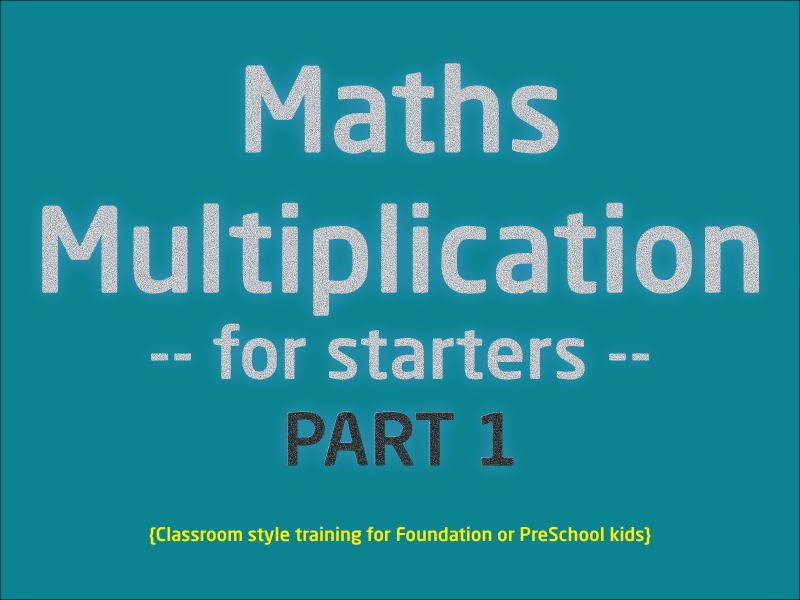### Multiplication for Starters

This lesson is split into 2 partsPart 1 discuss about the basics of multiplication and how new students should approach it.Part 2 shows visual examples plus multiplication table fo...

2 videos
Basic difficulty
Foundation/Maths
0 0 0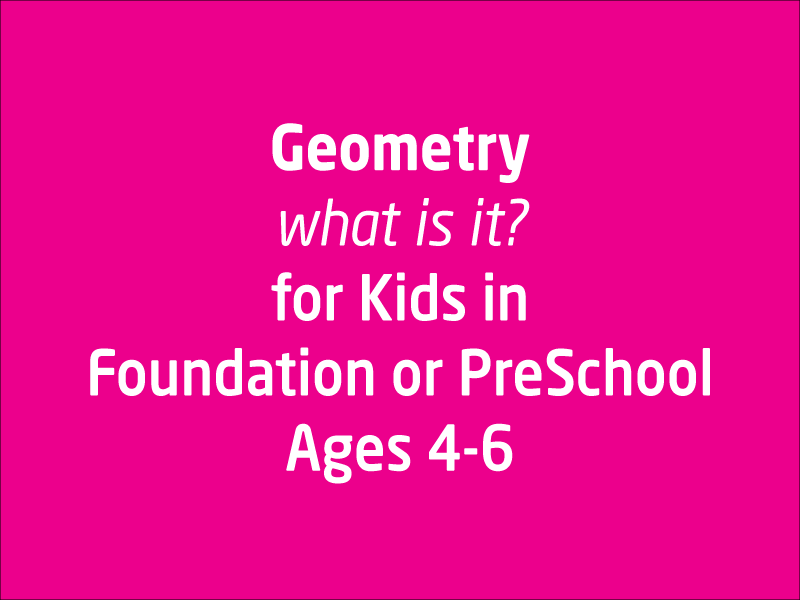### Geometry for Foundation and PreSchoolers

This lesson is not about understanding each and every concept covered in Geometry, because that's a vast and big subject in itself. However for little ones it gives them an opp...

1 videos
Basic difficulty
Foundation/Maths
0 0 0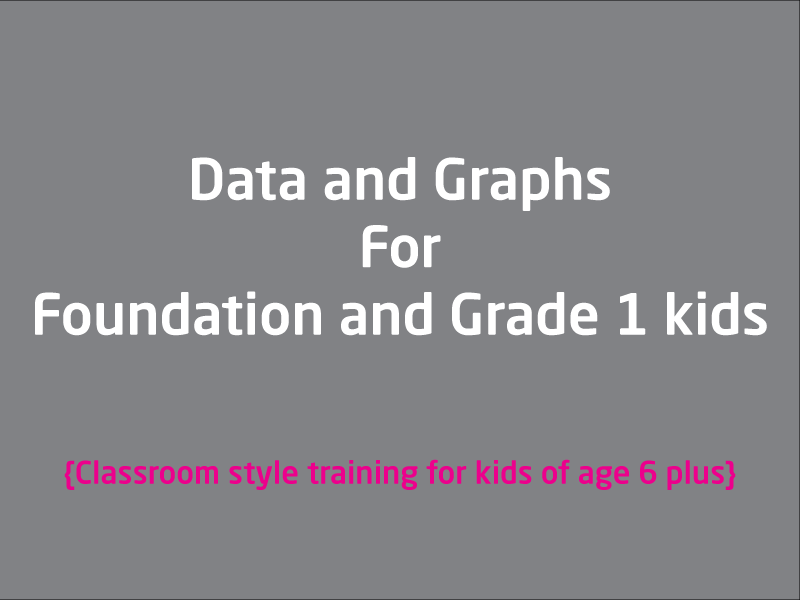### Introduction to Data and Graph

For kids aged 6+, this lesson can be very useful if they are just starting off to understand what Data is and What graph means. This is a live recorded classroom style lesson, send...

1 videos
Basic difficulty
Foundation/Maths
0 0 0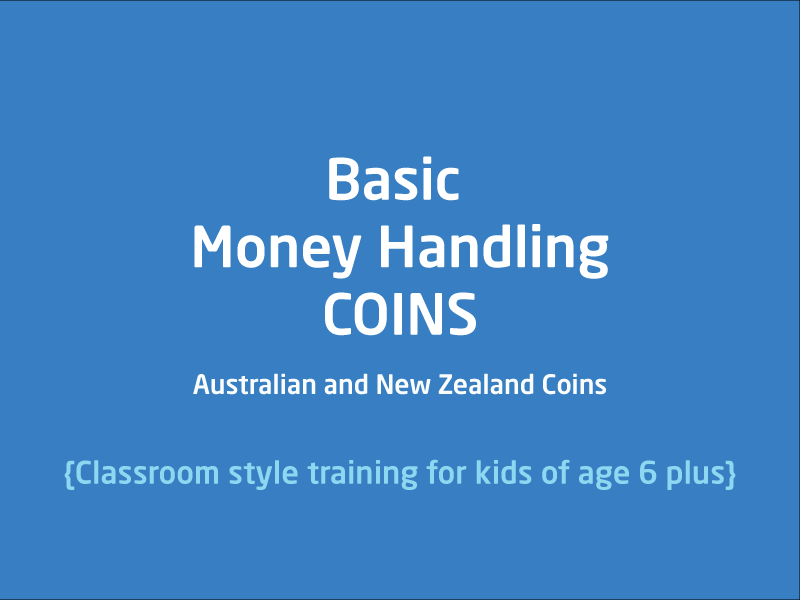### Coins

This lesson is for kids who are starting off with handling money. This lesson use Australian and New Zealand coins in examples that illustrates addition, subtraction, multiplying a...

1 videos
Basic difficulty
Foundation/Maths
0 0 0